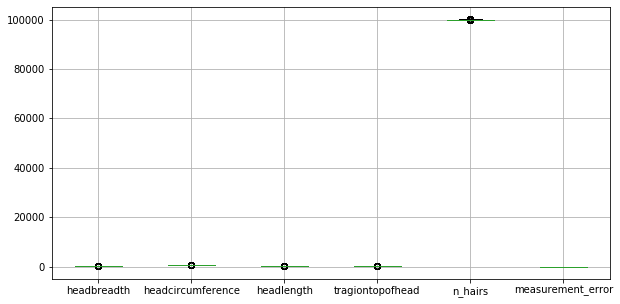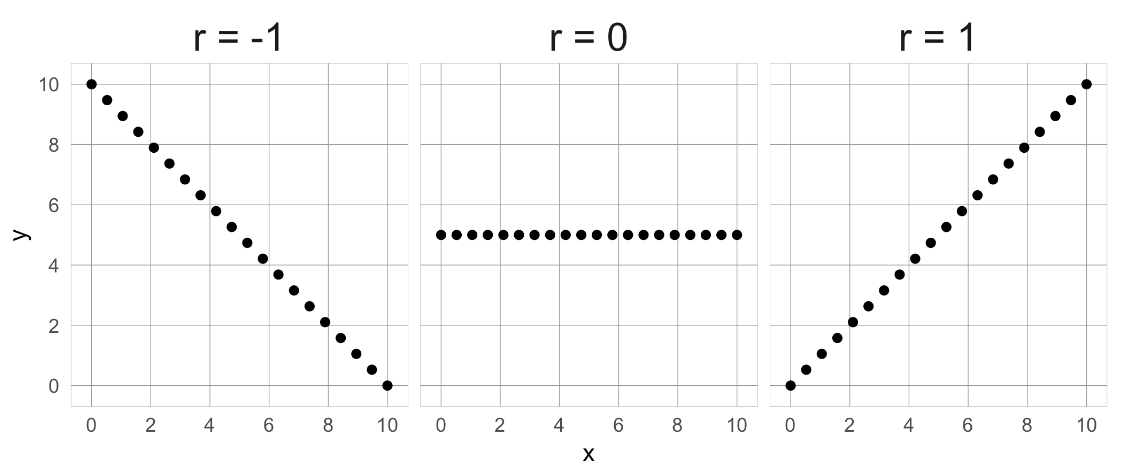import pandas as pd
import numpy as np
import matplotlib.pyplot as plt
import seaborn as sns

plt.rcParams['figure.figsize'] = (7, 7)


## The curse of dimensionality

### Train - test split

In this chapter, you will keep working with the ANSUR dataset. Before you can build a model on your dataset, you should first decide on which feature you want to predict. In this case, you're trying to predict gender.

You need to extract the column holding this feature from the dataset and then split the data into a training and test set. The training set will be used to train the model and the test set will be used to check its performance on unseen data.

ansur_male = pd.read_csv('./dataset/ANSUR_II_MALE.csv')

ansur_df = pd.concat([ansur_male, ansur_female])
# unused columns in the dataset
unused = ['Branch', 'Component', 'BMI_class', 'Height_class', 'BMI', 'weight_kg', 'stature_m']

# Drop the non-numeric columns from df
ansur_df.drop(unused, axis=1, inplace=True)

from sklearn.model_selection import train_test_split

# Select the Gender column as the feature to be predict (y)
y = ansur_df['Gender']

# Remove the Gender column to create the training data
X = ansur_df.drop('Gender', axis=1)

# Perform a 70% train and 30% test data split
X_train, X_test, y_train, y_test = train_test_split(X, y, test_size=0.3)

print("{} rows in test set vs. {} in training set. {} Features.".format(
X_test.shape, X_train.shape, X_test.shape
))

1821 rows in test set vs. 4247 in training set. 91 Features.


### Fitting and testing the model

In the previous exercise, you split the dataset into X_train, X_test, y_train, and y_test. These datasets have been pre-loaded for you. You'll now create a support vector machine classifier model (SVC()) and fit that to the training data. You'll then calculate the accuracy on both the test and training set to detect overfitting.

from sklearn.svm import SVC
from sklearn.metrics import accuracy_score

# Create an instance of the Support Vector Classification class
svc = SVC()

# Fit the model to the training data
svc.fit(X_train, y_train)

# Calculate accuracy scores on both train and test data
accuracy_train = accuracy_score(y_train, svc.predict(X_train))
accuracy_test = accuracy_score(y_test, svc.predict(X_test))

print("{0:.1%} accuracy on test set vs. {1:.1%} on training set".format(accuracy_test,
accuracy_train))

99.0% accuracy on test set vs. 99.0% on training set


Current data doesn't show overfitting. But example in datacamp shows overfitting from dataset.

ansur_df_overfit = pd.read_csv('./dataset/ansur_overfit.csv')

# Select the Gender column as the feature to be predict (y)
y = ansur_df_overfit['Gender']

# Remove the Gender column to create the training data
X = ansur_df_overfit.drop('Gender', axis=1)

# Perform a 70% train and 30% test data split
X_train, X_test, y_train, y_test = train_test_split(X, y, test_size=0.3)

print("{} rows in test set vs. {} in training set. {} Features.".format(
X_test.shape, X_train.shape, X_test.shape
))

# Create an instance of the Support Vector Classification class
svc = SVC()

# Fit the model to the training data
svc.fit(X_train, y_train)

# Calculate accuracy scores on both train and test data
accuracy_train = accuracy_score(y_train, svc.predict(X_train))
accuracy_test = accuracy_score(y_test, svc.predict(X_test))

print("{0:.1%} accuracy on test set vs. {1:.1%} on training set".format(accuracy_test,
accuracy_train))

300 rows in test set vs. 700 in training set. 91 Features.
91.3% accuracy on test set vs. 94.7% on training set


### Accuracy after dimensionality reduction

You'll reduce the overfit with the help of dimensionality reduction. In this case, you'll apply a rather drastic form of dimensionality reduction by only selecting a single column that has some good information to distinguish between genders. You'll repeat the train-test split, model fit and prediction steps to compare the accuracy on test vs. training data.

X = ansur_df_overfit[['neckcircumferencebase']]

# SPlit the data, instantiate a classifier and fit the data
X_train, X_test, y_train, y_test = train_test_split(X, y, test_size=0.3)
svc = SVC()
svc.fit(X_train, y_train)

# Calculate accuracy scores on both train and test data
accuracy_train = accuracy_score(y_train, svc.predict(X_train))
accuracy_test = accuracy_score(y_test, svc.predict(X_test))

print("{0:.1%} accuracy on test set vs. {1:.1%} on training set".format(accuracy_test,
accuracy_train))

92.7% accuracy on test set vs. 95.0% on training set


On the full dataset the model is rubbish but with a single feature we can make good predictions? This is an example of the curse of dimensionality! The model badly overfits when we feed it too many features. It overlooks that neck circumference by itself is pretty different for males and females.

## Features with missing values or little variance

### Finding a good variance threshold

You'll be working on a slightly modified subsample of the ANSUR dataset with just head measurements

head_df = pd.read_csv('./dataset/head_df.csv')

fig, ax = plt.subplots(figsize=(10, 5));normalized_df = head_df / head_df.mean()

# Print the variances of the normalized data
print(normalized_df.var())

fig, ax = plt.subplots(figsize=(10, 10));
normalized_df.boxplot(ax=ax);

headbreadth          1.678952e-03
n_hairs              1.002552e-08
measurement_error    3.231707e-27
dtype: float64### Features with low variance

In the previous exercise you established that 0.001 is a good threshold to filter out low variance features in head_df after normalization. Now use the VarianceThreshold feature selector to remove these features.

from sklearn.feature_selection import VarianceThreshold

# Create a VarianceThreshold feature selector
sel = VarianceThreshold(threshold=0.001)

# Fit the selector to normalized head_df

# Apply the mask to create a reduced dataframe

print("Dimensionality reduced from {} to {}".format(head_df.shape, reduced_df.shape))

Dimensionality reduced from 6 to 4


### Removing features with many missing values

You'll apply feature selection on the Boston Public Schools dataset which has been pre-loaded as school_df. Calculate the missing value ratio per feature and then create a mask to remove features with many missing values.

school_df = pd.read_csv('./dataset/Public_Schools2.csv')

mask = school_df.isna().sum() / len(school_df) < 0.5

# Create a reduced dataset by applying the mask

print(school_df.shape)
print(reduced_df.shape)

(131, 21)
(131, 19)


## Pairwise correlation

• Correlation coefficient (r)### Visualizing the correlation matrix

Reading the correlation matrix of ansur_df in its raw, numeric format doesn't allow us to get a quick overview. Let's improve this by removing redundant values and visualizing the matrix using seaborn.

ansur_df_sample = ansur_df[['elbowrestheight', 'wristcircumference', 'anklecircumference',
'buttockheight', 'crotchheight']]
ansur_df_sample.columns = ['Elbow rest height', 'Wrist circumference',
'Ankle circumference', 'Buttock height', 'Crotch height']

Elbow rest height Wrist circumference Ankle circumference Buttock height Crotch height
0 247 175 222 882 877
1 232 167 220 870 851
2 237 180 230 901 854
3 272 176 230 821 769
4 188 188 247 1080 1014
corr = ansur_df_sample.corr()

cmap = sns.diverging_palette(h_neg=10, h_pos=240, as_cmap=True)

# Draw the heatmap
sns.heatmap(corr,  cmap=cmap, center=0, linewidths=1, annot=True, fmt=".2f");mask = np.triu(np.ones_like(corr, dtype=bool))## Removing highly correlated features

### Filtering out highly correlated features

You're going to automate the removal of highly correlated features in the numeric ANSUR dataset. You'll calculate the correlation matrix and filter out columns that have a correlation coefficient of more than 0.95 or less than -0.95.

Since each correlation coefficient occurs twice in the matrix (correlation of A to B equals correlation of B to A) you'll want to ignore half of the correlation matrix so that only one of the two correlated features is removed. Use a mask trick for this purpose.

ansur_male = pd.read_csv('./dataset/ANSUR_II_MALE.csv')
ansur_df = ansur_male

corr_matrix = ansur_df.corr().abs()

# Create a True/False mask and apply it

# List column names of highly correlated features (r > 0.95)
to_drop = [c for c in tri_df.columns if any(tri_df[c] > 0.95)]

# Drop the features in the to_drop list
reduced_df = ansur_df.drop(to_drop, axis=1)

print("The reduced dataframe has {} columns.".format(reduced_df.shape))

The reduced dataframe has 88 columns.


### Nuclear energy and pool drownings

The dataset that has been pre-loaded for you as weird_df contains actual data provided by the US Centers for Disease Control & Prevention and Department of Energy.

Let's see if we can find a pattern.

weird_df = pd.read_csv('./dataset/weird_df.csv')

print(weird_df.head())

   pool_drownings  nuclear_energy
0             421           728.3
1             465           753.9
2             494           768.8
3             538           780.1
4             430           763.7

sns.scatterplot(x='nuclear_energy', y='pool_drownings', data=weird_df);print(weird_df.corr())

                pool_drownings  nuclear_energy
pool_drownings        1.000000        0.901179
nuclear_energy        0.901179        1.000000


But correlation of the data does not imply causation.